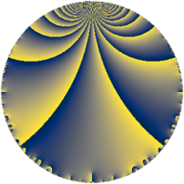# Properties

 Label 245.4.eLevel $245$ Weight $4$ Character orbit 245.e Rep. character $\chi_{245}(116,\cdot)$ Character field $\Q(\zeta_{3})$ Dimension $80$ Newform subspaces $17$ Sturm bound $112$ Trace bound $3$

# Related objects

## Defining parameters

 Level: $$N$$ $$=$$ $$245 = 5 \cdot 7^{2}$$ Weight: $$k$$ $$=$$ $$4$$ Character orbit: $$[\chi]$$ $$=$$ 245.e (of order $$3$$ and degree $$2$$) Character conductor: $$\operatorname{cond}(\chi)$$ $$=$$ $$7$$ Character field: $$\Q(\zeta_{3})$$ Newform subspaces: $$17$$ Sturm bound: $$112$$ Trace bound: $$3$$ Distinguishing $$T_p$$: $$2$$, $$3$$

## Dimensions

The following table gives the dimensions of various subspaces of $$M_{4}(245, [\chi])$$.

Total New Old
Modular forms 184 80 104
Cusp forms 152 80 72
Eisenstein series 32 0 32

## Trace form

 $$80 q - 4 q^{2} - 12 q^{3} - 140 q^{4} - 10 q^{5} - 8 q^{6} - 72 q^{8} - 406 q^{9} + O(q^{10})$$ $$80 q - 4 q^{2} - 12 q^{3} - 140 q^{4} - 10 q^{5} - 8 q^{6} - 72 q^{8} - 406 q^{9} - 20 q^{10} + 46 q^{11} - 34 q^{12} - 48 q^{13} - 80 q^{15} - 752 q^{16} - 132 q^{17} - 138 q^{18} + 18 q^{19} + 280 q^{20} - 984 q^{22} + 412 q^{23} + 728 q^{24} - 1000 q^{25} + 278 q^{26} + 840 q^{27} + 636 q^{29} - 140 q^{30} + 168 q^{31} + 224 q^{32} - 320 q^{33} - 824 q^{34} + 1040 q^{36} + 180 q^{37} + 96 q^{38} + 632 q^{39} - 240 q^{40} + 384 q^{41} - 424 q^{43} + 1150 q^{44} - 600 q^{45} + 578 q^{46} - 624 q^{47} - 164 q^{48} + 200 q^{50} + 88 q^{51} - 668 q^{52} + 20 q^{53} - 72 q^{54} + 640 q^{55} + 408 q^{57} + 3334 q^{58} + 1188 q^{59} + 1130 q^{60} + 2434 q^{61} - 360 q^{62} + 7784 q^{64} + 430 q^{65} + 1988 q^{66} - 2716 q^{67} - 4696 q^{68} - 3324 q^{69} - 6224 q^{71} - 2650 q^{72} - 1156 q^{73} - 3798 q^{74} - 300 q^{75} - 852 q^{76} + 15280 q^{78} - 3700 q^{79} - 1280 q^{80} - 5412 q^{81} + 2050 q^{82} + 5280 q^{83} + 2000 q^{85} + 3144 q^{86} + 1684 q^{87} + 13352 q^{88} + 1778 q^{89} - 540 q^{90} - 13780 q^{92} - 4332 q^{93} - 2210 q^{94} + 320 q^{95} + 6404 q^{96} - 1744 q^{97} - 22516 q^{99} + O(q^{100})$$

## Decomposition of $$S_{4}^{\mathrm{new}}(245, [\chi])$$ into newform subspaces

Label Dim $A$ Field CM Traces $q$-expansion
$a_{2}$ $a_{3}$ $a_{5}$ $a_{7}$
245.4.e.a $2$ $14.455$ $$\Q(\sqrt{-3})$$ None $$-3$$ $$-2$$ $$5$$ $$0$$ $$q-3\zeta_{6}q^{2}+(-2+2\zeta_{6})q^{3}+(-1+\zeta_{6})q^{4}+\cdots$$
245.4.e.b $2$ $14.455$ $$\Q(\sqrt{-3})$$ None $$-1$$ $$-8$$ $$-5$$ $$0$$ $$q-\zeta_{6}q^{2}+(-8+8\zeta_{6})q^{3}+(7-7\zeta_{6})q^{4}+\cdots$$
245.4.e.c $2$ $14.455$ $$\Q(\sqrt{-3})$$ None $$-1$$ $$-6$$ $$5$$ $$0$$ $$q-\zeta_{6}q^{2}+(-6+6\zeta_{6})q^{3}+(7-7\zeta_{6})q^{4}+\cdots$$
245.4.e.d $2$ $14.455$ $$\Q(\sqrt{-3})$$ None $$-1$$ $$6$$ $$-5$$ $$0$$ $$q-\zeta_{6}q^{2}+(6-6\zeta_{6})q^{3}+(7-7\zeta_{6})q^{4}+\cdots$$
245.4.e.e $2$ $14.455$ $$\Q(\sqrt{-3})$$ None $$-1$$ $$8$$ $$5$$ $$0$$ $$q-\zeta_{6}q^{2}+(8-8\zeta_{6})q^{3}+(7-7\zeta_{6})q^{4}+\cdots$$
245.4.e.f $2$ $14.455$ $$\Q(\sqrt{-3})$$ None $$4$$ $$-2$$ $$5$$ $$0$$ $$q+4\zeta_{6}q^{2}+(-2+2\zeta_{6})q^{3}+(-8+8\zeta_{6})q^{4}+\cdots$$
245.4.e.g $2$ $14.455$ $$\Q(\sqrt{-3})$$ None $$4$$ $$2$$ $$-5$$ $$0$$ $$q+4\zeta_{6}q^{2}+(2-2\zeta_{6})q^{3}+(-8+8\zeta_{6})q^{4}+\cdots$$
245.4.e.h $4$ $14.455$ $$\Q(\sqrt{2}, \sqrt{-3})$$ None $$-8$$ $$-2$$ $$10$$ $$0$$ $$q+(-\beta _{1}+4\beta _{2}-\beta _{3})q^{2}+(-1-4\beta _{1}+\cdots)q^{3}+\cdots$$
245.4.e.i $4$ $14.455$ $$\Q(\sqrt{2}, \sqrt{-3})$$ None $$-8$$ $$2$$ $$-10$$ $$0$$ $$q+(-\beta _{1}+4\beta _{2}-\beta _{3})q^{2}+(1+4\beta _{1}+\cdots)q^{3}+\cdots$$
245.4.e.j $4$ $14.455$ $$\Q(\sqrt{-3}, \sqrt{11})$$ None $$-2$$ $$-10$$ $$-10$$ $$0$$ $$q+(-1+\beta _{1}-\beta _{2})q^{2}+5\beta _{2}q^{3}+(-2\beta _{1}+\cdots)q^{4}+\cdots$$
245.4.e.k $4$ $14.455$ $$\Q(\sqrt{-3}, \sqrt{11})$$ None $$-2$$ $$10$$ $$10$$ $$0$$ $$q+(-1+\beta _{1}-\beta _{2})q^{2}-5\beta _{2}q^{3}+(-2\beta _{1}+\cdots)q^{4}+\cdots$$
245.4.e.l $4$ $14.455$ $$\Q(\sqrt{2}, \sqrt{-3})$$ None $$6$$ $$-2$$ $$10$$ $$0$$ $$q+(\beta _{1}-3\beta _{2}+\beta _{3})q^{2}+(-1-3\beta _{1}+\cdots)q^{3}+\cdots$$
245.4.e.m $6$ $14.455$ 6.0.5567659200.1 None $$3$$ $$-2$$ $$-15$$ $$0$$ $$q+(1-\beta _{1}-\beta _{2}-\beta _{3})q^{2}+(\beta _{1}-\beta _{3}+\cdots)q^{3}+\cdots$$
245.4.e.n $6$ $14.455$ 6.0.5567659200.1 None $$3$$ $$2$$ $$15$$ $$0$$ $$q+(1-\beta _{1}-\beta _{2}-\beta _{3})q^{2}+(-\beta _{1}+\beta _{3}+\cdots)q^{3}+\cdots$$
245.4.e.o $10$ $14.455$ $$\mathbb{Q}[x]/(x^{10} - \cdots)$$ None $$-1$$ $$-8$$ $$-25$$ $$0$$ $$q+(\beta _{1}-\beta _{2})q^{2}+(-\beta _{3}-\beta _{5}-2\beta _{7}+\cdots)q^{3}+\cdots$$
245.4.e.p $12$ $14.455$ $$\mathbb{Q}[x]/(x^{12} - \cdots)$$ None $$2$$ $$-16$$ $$30$$ $$0$$ $$q-\beta _{5}q^{2}+(-3-3\beta _{3}+\beta _{7}+\beta _{9})q^{3}+\cdots$$
245.4.e.q $12$ $14.455$ $$\mathbb{Q}[x]/(x^{12} - \cdots)$$ None $$2$$ $$16$$ $$-30$$ $$0$$ $$q-\beta _{5}q^{2}+(3+3\beta _{3}-\beta _{7}-\beta _{9})q^{3}+\cdots$$

## Decomposition of $$S_{4}^{\mathrm{old}}(245, [\chi])$$ into lower level spaces

$$S_{4}^{\mathrm{old}}(245, [\chi]) \cong$$ $$S_{4}^{\mathrm{new}}(7, [\chi])$$$$^{\oplus 4}$$$$\oplus$$$$S_{4}^{\mathrm{new}}(35, [\chi])$$$$^{\oplus 2}$$$$\oplus$$$$S_{4}^{\mathrm{new}}(49, [\chi])$$$$^{\oplus 2}$$